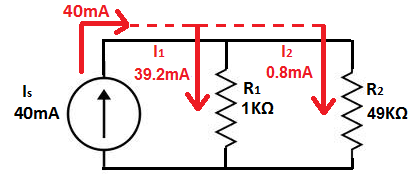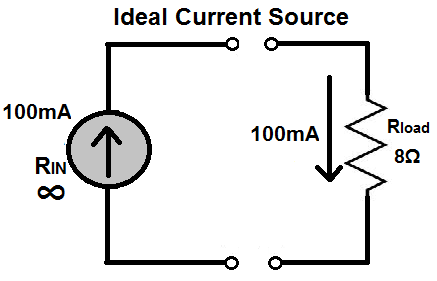﻿ What is a Constant Current Source? ﻿# What is a Constant Current Source?A constant current source is a power source which provides a constant current to a load, even despite changes and variance in load resistance.

In other words, the current which a constant current source provides is steady, even if the resistance of the load varies.

A constant current source is, thus, a very valuable component because it can supply steady current even if there are changes in resistance, even a wide variance in the resistance. This comes in use when a circuit needs a steady current supply, without fluctuations.

The graph below represents the current which comes from a constant current source.You can see that the current is constant all throughout despite changes in voltage or resistance.

### How Does A Constant Current Source Work?

A constant current source is a power generator whose internal resistance is very high compared with the load resistance it is giving power to. Because its internal resistance is so high, it can supply a constant current to a load whose resistance value varies, even over a wide range.

Thus, a constant current source follows the rules of current division. Being that it has very high internal resistance and the load resistance is much lower, current takes the path of least resistance, flowing out of the (high internal resistance) current source and into the load resistance, since it is of much lower resistance.

If you know current division, current takes the path of least resistance. Look at the following current divider circuit below:Notice how this current source, shown above, supplies 40mA of total current from out of it. The majority of this 40mA of current takes the path of least resistance, the 5KΩ resistor, and the other 10mA of current goes through the larger resistance, 15KΩ.

Now let's increase the difference in resistances between the two resistors, so that the current division is even more pronounce.Now that one resistor is 1KΩ and the other resistor is 49KΩ, the vast majority of the current goes through the 1KΩ resistor. Very little current goes through the 49KΩ, because it is such a great resistance.

Now let's increase the resistance again. The below current source represents a current source which has infinite internal resistance.Because the resistance is infinite and the load is only 8Ω, most of the current goes through the 8Ω resistor, which is the path of least resistance. Again, current always take the path of least resistance. Since the load has infinite internal resistance, current will always seek to escape from it to a lower resistance path.

This is how constant current sources work.

### Constant Current Source Circuit

A constant current source circuit is just a constant current source connected to the load which it powers.This load above will have a constant current of 50mA supplied to it regardless of whether the load resistance varies.

Related Resources